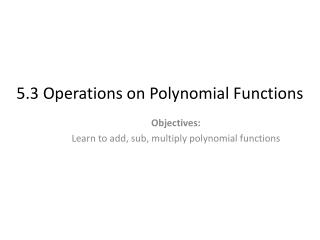Download Presentation5.3 Operations on Polynomial Functions

# 5.3 Operations on Polynomial Functions - PowerPoint PPT Presentation

5.3 Operations on Polynomial Functions. Objectives: Learn to add, sub, multiply polynomial functions. Example 1. Add (5x 2 + 3x + 4) + (3x 2 + 5). = 8x 2. + 3x. + 9. Example 2. Add. (2x 4 – 5x 2 + 4x + 5) + (5x 4 + 7x 3 – 2x 2 – 2x). 2x 4 + 0x 3 – 5x 2 + 4x + 5.I am the owner, or an agent authorized to act on behalf of the owner, of the copyrighted work described.
Download Presentation## 5.3 Operations on Polynomial Functions

Download Policy: Content on the Website is provided to you AS IS for your information and personal use and may not be sold / licensed / shared on other websites without getting consent from its author.While downloading, if for some reason you are not able to download a presentation, the publisher may have deleted the file from their server.

- - - - - - - - - - - - - - - - - - - - - - - - - - E N D - - - - - - - - - - - - - - - - - - - - - - - - - -
Presentation Transcript
1. 5.3 Operations on Polynomial Functions Objectives: Learn to add, sub, multiply polynomial functions

2. Example 1 Add (5x2 + 3x + 4) + (3x2 + 5) = 8x2 + 3x + 9

3. Example 2 Add. (2x4 – 5x2 + 4x + 5) + (5x4 + 7x3 – 2x2 – 2x) 2x4 + 0x3 – 5x2 + 4x + 5 5x4 + 7x3 – 2x2 – 2x + 0 7x4 + 7x3 – 7x2 + 2x + 5

4. Practice Add. 1) (-2m3 – 5m2 – 2m – 4) + (m4 – 6m2 + 7m – 10)

5. Example 1 Subtract. (5x2 + 3x - 2) - (2x2 + 1) = 5x2 + 3x - 2 - 2x2 - 1 = 3x2 + 3x - 3

6. Practice Subtract. 1) (5x4 + 4) – (2x2 – 1) 2) (-7m3 + 2m + 4) – (-2m3 – 4)

7. Example 2 Subtract. (8x3 + 6x2 – 3x + 5) – (5x3 – 3x2 + 2x – 4) 8x3 + 6x2 – 3x + 5 -5x3 + 3x2 - 2x + 4 3x3 + 9x2 - 5x + 9

8. Practice Subtract. 1) (-2m3 – 5m2 – 2m – 4) - (m4 – 6m2 + 7m – 10)

9. Example 1 Multiply. (x + 1)(x2 + x + 1) = (x)(x2 + x + 1) + (1)(x2 + x + 1) = (x3 + x2 + x) + (x2 + x + 1) = x3 + 2x2 + 2x + 1

10. Example 2 Multiply. (2x + 3)(x2 + 4x + 5) = (2x)(x2 + 4x + 5) + (3)(x2 + 4x + 5) = (2x3 + 8x2 + 10x) + (3x2 + 12x + 15) = 2x3 + 11x2 + 22x + 15

11. Practice Multiply. 1) (x2 + 5)(x2 + 3x – 4)

12. Example 3 Multiply. (5x2 + 3x + 2)(3x2 + 2x – 1) 15x4 + 10x3 – 5x2 9x3 – 3x + 6x2 6x2 + 4x - 2 15x4 + 19x3 + 7x2 + x - 2

13. Practice Multiply. 1) (3x2 – 2x + 4)(x2 + 5x + 1)

14. Assignment • Pg349, 350 #3-33 multiples of 3, 59